# Torsional stresses

In this tutorial we examine stresses induced when a cylindrical component, such as a machine shaft, is subjected to torsion generated by a torque load.  This analysis is valid only for elastic strains (Hooke's Law).

### Shear stresses in torsion

Consider the cylindrical section with radius R and length L shown below.The rear face of the section is fixed and an anti-clockwise torque T is applied to the fromt face.  There is an opposong reaction torque on the fixed rear face.

We consider an "elemental tube" inside the cylinder at radius r and width dr.  Undere the action of T the element twists and experiences shear strain γ.  Correspondingly, the front face of the element twists through angle θ.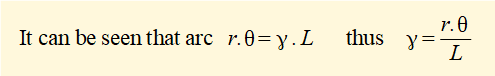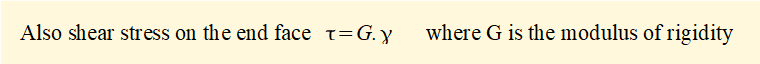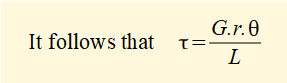The diagram shows the shear stress τ on the end face varies linearlly with radius r.  τmax is at the outer surface of the cylinder.

For bending we established a relation between bending moment M and normal stress σ.  We now do likewise of torque T and shear stress τ.

By equating the torque induced on the face of the cylinder by the shear stress to the applied torque it is shown that: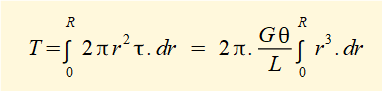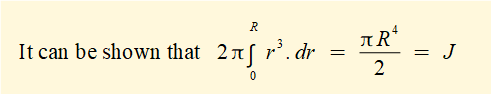where J is the second polar moment of area about the central axis of a cylinder.  Units of J are m4.

Note that the above integral is not the general definition of J, which is the double integral: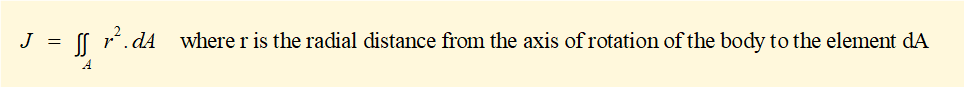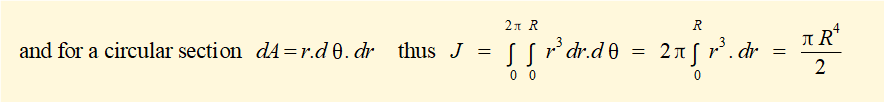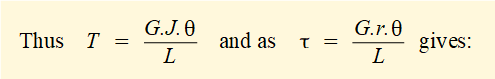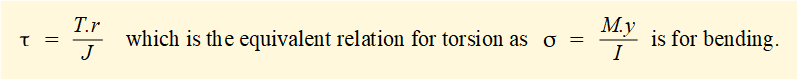It can also be shown that the above relation applies to a hollow cylindrical shaft.  For a hollow cylinder: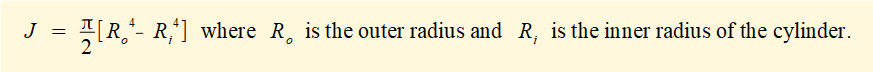### Normal stresses in torsion

Consider a square element on the outer surface of a cylinder such that the top and bottom sides of the element are parallel to the axis of the cylinder.  The cylinder is sbject to a torsional load.Stresses τ on the sides of this element are pure shear (no normal stresse).  This state is represented by the blue τ axis of Mohr's circle below which shows that  τ on the element is the maximum shear stress.

Now consider the element rotated 45° such that the sides of the element are parallel with lines ac and bd.  This state is represented by the red σ axis of Mohr's circle (remembering that the rotation of  the circle diameter is twice that of the stress element) which shows that σ1 and σ2 are principal stresses equal in magnitude to  τmax.  Tensile stress  σ1 acts normal to plane bd and compressive stress σ2 normal to plane ac.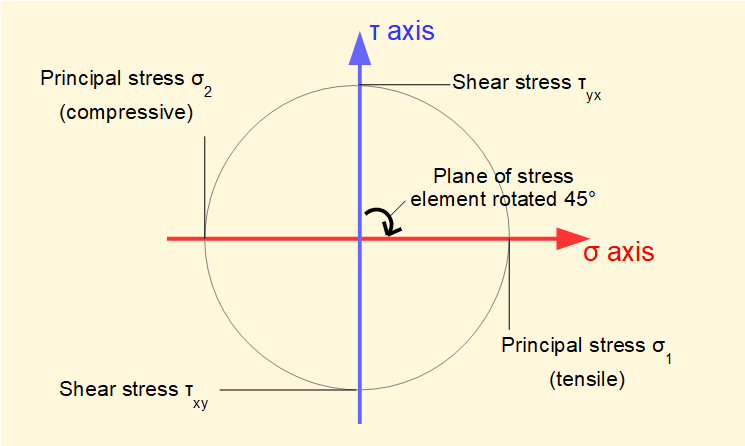A consequence of the principal stresses being on these planes is that a component subject to torsion and made from a brittle material  will generally fail in tension at an angle of 45° to the longitudinal axis.

### Tutorials - mechanical vibrations

###### Forced vibrations with damping

Alistair's tutorials 2019